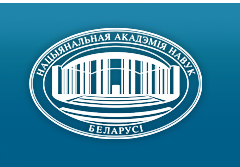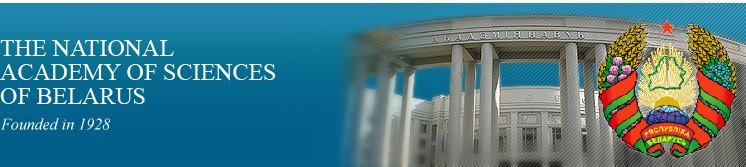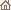Home News About Academy Academy Activities Academy Membership Organizations Publications Conferences Contacts Site Map

# Proc. of the Natl. Academy of Sciences of Belarus, Ser. Phys.-Math. Sci., 2005, No.3/ Publications / Scientific Journals

Proc. of the Natl. Academy of Sciences of Belarus, Ser. Phys.-Math. Sci., 2005, No.3Proceedings of the National Academy of Sciences of Belarus (Vestsi Natsiyanalnai Akademii Navuk Belarusi) SERIES OF PHYSICAL-MATHEMATICAL SCIENCESPublished by Belaruskaya Navuka Publishing House, Minsk, Republic of Belarus

### Number 3, 2005## CONTENTS

### MATHEMATICS

Gorokhovik V. V.
On second-order local minimum conditions for smooth optimization problems with abstract constraints. pp. 5--12

Summary: For the problem of minimizing a twice Freshet differentiable function f : X ® R over an abstract set Q Ì X we supplement known second order necessary local minimum conditions with a new one which should be satisfied by nonzero second order asymptotic tangent vectors to Q. We also prove that in finite-dimensional settings the collection consisting of strengthened (known and new) necessary conditions is sufficient for local strict minimum in the problem under consideration.

Zabrejko P.P., Tykoun A. S.
The Conley index and guiding functions method in the theory of bounded solutions of differential equations. pp. 13--18

Summary: Theorems which established relationship between the Conley index and method of guiding functions for autonomous systems of differential equations are proved. Theorems give the conditions of existence of the Conley index and amplify the Krasnoselski - Perov theorems.

Sarazhinsky D. S.
Quasidifferentiability of the class of eigenelements of the Perron--Frobenius operators. pp. 19--24

Summary: The Perron--Frobenius operator generated by the shift mapping on a space of sequences with a finite number of values is considered. It is supposed that the dependence of the corresponding potential function on the coordinate number decreases with a power rate. In this case some kind of Taylor expansion for eigenelements with respect to potential function is constructed.

Tsurko V. A.
Iteration method for finding the difference solution of the two-dimensional hyperbolic equations of the second order with discontinuous coefficients. pp. 25--31

Summary: Difference schemes for the linear hyperbolic equations are considered. Stability of the symmetric difference schemes with respect to the initial data is proved. Effective iteration method for finding the difference solution of the given equation is proposed and investigated.

Bodyagin D. A.
Inhomogeneous approximations and lower bounds of Hausdorff dimension. pp. 32--36

Summary: The exact lower bound for Hausdorff dimension of sets associated with inhomogeneous Diophantine approximation on non-degenerate curves is found.

Lepeyev A. N.
Stochastic differential inclusions with unbounded diffusion coefficient. pp. 37--43

Summary: One-dimensional homogeneous stochastic differential inclusions without drift with Borel-measurable right sides are considered. The right sides, construction of the several selections and existence conditions for the solutions of the inclusions with locally unbounded right sides are investigated. The main result is a necessary and sufficient condition for the existence of a explicit weak solution of inclusions for every initial distribution.

Zuev N. M., Koshkin Ja. B.
Non-uniform estimation of the convergence rate of the distribution of the maximum of sums of m-dependent random variables. pp. 44--49

Summary: The estimation for a stationary in the strong sence random process with bounded third moment is obtained. Estimations for bounded random variables, random variables with bounded moment of order higher than three and random variables satisfying the generalized Kramer condition are established.

Rozin E. B.
The associated solutions of system of the equations in the differentials containing jumping random process. pp. 50--55

Summary: Solutions of the system of equations in the differentials are investigated. It is shown the convergence of the solution to the corresponding solution of the system of stochastic differential equations with the jumping process.

Myaleshka I. N.
The approximate solution with the logarithms of one Dirichlet problem for the circle. pp. 56--59

Summary: It has been obtained an approximate expression representing the solution of one Dirichlet boundary-value problem for Poisson equation in logarithms in a circle.

### PHYSICS

Bogush A. A.
On the quantum vector parameters of the q-deformed supergroup. pp. 60--66

Summary: The basic relations in the theory of the quantum (q-deformed) supergroup GLq(2) are presented in the new form, being symmetrical with respect to the quantum deformation parameters q1/2 and q-1/2. The description of this group in terms of the introduced four-dimensional quantum vector parameters which obey the simple composition rules is given.

Voitikova M. V., Voitovich A. P.
Wavelet analysis of the event-related potential in electroencephalo-graphic research. pp. 67--72

Summary: The article deals with 5-level discrete wavelet transform in investigation of children brain dynamics and event-related potential, which provide evidence of direct linkage between cognitive events, electrochemical brain events and genetic predisposition to alcoholism. Wavelet transform was applied to electroencephalographic data (EEC), received during the simple task of comparison of two images. The wavelet-coefficients are rejected in the time interval outside of event-related response. Thus allocation of weak wave component on a background of spontaneous variations EEG was carried out, which amplitude and latencies indicate on genetic predisposition to alcoholism disease.

Chernichenko Yu. D.
Solving of the relativistic quasipotential equation for a sum of nonlocal separable quasipotentials. pp. 73--80

Summary: Solving of the finite-difference quasipotential equation involving a total quasipotential simulating the interaction of two relativistic spinless particles of unequal masses is obtained. The total interaction consisting of the superposition of a local quasipotential and a sum of nonlocal separable quasipotentials is the spherically symmetric quasipotential and it admits one true bound state. The problem is investigated within the relativistic quasipotential approach to quantum field theory. Explicit expressions are obtained for the additionals of the phase shift and their properties are investigated, the conditions under which bound states may exist are determined and the Levinson theorem is generalized.

Tuniak U. M.
Brans--Dicke scalar field and Poincare' gauge theory of gravitation in the Riemann--Cartan space. pp. 81--84

Summary: The field equations of the generalized Poincare gauge theory of gravitation in the Riemann--Cartan space were obtained at the variational principle base, the theory is the natural unification of the Poincare gauge theory of gravitation and scalar-tensor Brans--Dicke theory. The conditions are determined due to which the Brans--Dicke theory spherically-symmetric static vacuum solution is this theory vacuum torsion-tree field.

Shishkina T. V.
Modified leading logarithm method for calculation of radiative effects in deep inelastic electroweak interaction of polarized particles. pp. 85--91

Summary: Modified method of leading logarithm approximation including contribution of radiative correction of collinear photon bremsstrahlung is proposed and used for calculation of deep inelastic scattering of polarized particles. The analytic Lorentz-invariant expressions are presented. Radiative effect contribution to the differential cross sections of deep inelastic scattering of polarized leptons by polarized nucleons and asymmetries of different nature are calculated. The method accuracy is estimated. Increasing of new result precision is discovered.

Filippov V. V., Baldin V. A.
Normal modes in absorbing monoclinic crystals. pp. 92--95

Summary: Exact and simple approximate axpressions for the ellipticity, the angle x defining the polarization ellipse orientation, and refractive indices of normal modes are derived. Numerical results are presented for KGd(WO4)2 crystal doped with Nd3+ ions. It is shown the angle c is small and may be ignored in contrast to the ellipticity which can be noticeable despite the absorption of crystal is not large.

Zhestkov S. V.
On the existence of soliton solutions of multidimensional system coupled of high dispersive nonlinear Schroedinger equations. pp. 96--99

Summary: The one-soliton solution for common system of coupled nonlinear any order Schroedinger equations with derivatives of fourth order is constructed. The necessary and sufficient conditions of the existence of this solution axe obtained.

Karolik A. S., Sharando V. I.
Thermoelectric power due to dislocations in nickel. pp. 100--104

Summary: On measurements the thermoelectric power induced by tensile plastic deformation the change of thermoelectric power per unit of dislocation density DSd/Nd is fined for nickel (99.98%) at temperature close to the room one. The measured value is compared with the result of calculation. The calculation is performed by the partial-wave method on the assumption of the existence of localized electron states on the dislocations near the Fermi energy. Quite satisfactory agreement between measured and calculated values is obtained.

### INFORMATICS

Adutskevich E. V., Likhoded N. A., Sobolevsky P.I.
Parallelizing affine loop nests when distribution of operations over processors is given. pp. 105--111

Summary: The method for parallelizing affine loop nests in the case of given distribution of operations over processors is presented. The method takes into account dependence on outer loop indices and can be used for automated parallelizing programs.

Sadykhov R. Kh., Khomenko A. A.
Modified Delaunay triangulation algorithm with caching of triangle search. pp. 112--118

Summary: New modification of Delaunay triangulation algorithm is presented. This algorithm is based on Delaunay trian-gulation with dynamic caching of triangle search. The main peculiarities of this algorithm are: integer calculations, modified Delaunay condition check, iterations limit (while searching for the triangle). Special data structure is presented to hold triangulation results. The algorithm has been compared with two others and shown good performance results.

### ABSTRACTS OF DEPOSITED PAPERS

Melnikau A. I., Zaleski M. A.
Recognition of P4-structure of sunlike graph. p. 119

### SCIENTISTS OF BELARUS

Andrey Aleksandrovich Bogush (On the occasion of the 80th birthday). pp. 120--122
Click
here to read this article in Belarusian (Win 1251)

Fadey Fadeyevich Komarov (On the occasion of the 60th birthday). pp. 123--124
Click here to read this article in Russian (Win 1251)/ Publications / Scientific Journals / Back to Top of Page

Designed and maintained by Dr. Nikolai N. Kostyukovich. Last updated: November 2, 2005
Created with assistance of Dr. Ignatii I. Korsak
Copyright © 2005 The National Academy of Sciences of Belarus
Copyright © 2005 The Belaruskaya Navuka Publishing House